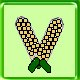Math problems

This page is used to construct a set of simple arithmetic problems. You can choose the number of problems presented, as well as the types of questions asked. Fill in all of the boxes and then hit the Submit button to generate the problems. Once generated you can print the sheet out for work offline, or you can answer the problems on the computer and have the results graded.

I have added an option to do numbers with digits past the decimal point. This changes the program in fundamental ways. If you find any errors with how this works, please drop me a note with the math problem that it gets wrong. It should only be a problem (if it is a problem) when you are solving the problem online. Send the note to Dave Regan

 Do you want addition questions? Can numbers to be added be negative? Can results be negative? Allow regrouping problems How many addition problems do you want? How large can the numbers be? Digits past the decimal point

#### Subtraction

 Do you want subtraction questions? Can numbers to be subtracted be negative? Can results be negative? Allow regrouping problems How many subtraction problems do you want? How large can the numbers be? Digits past the decimal point

#### Multiplication

 Do you want multiplication questions? Can numbers to be multiplied be negative? Can results be negative? How many multiplication problems do you want? How large can the numbers be? Digits past the decimal point. Note that the answer may have up to twice this many digits.

#### Division (no remainders)

 Do you want division questions? Can numbers to be divided be negative? Can results be negative? How many division problems do you want? How large can the numbers be?

#### Division (with remainders)

 Do you want division questions? Can numbers to be divided be negative? Can results be negative? How many division problems do you want? How large can the numbers be?

Answers are represented with an 'r' separating the
integer part of the answer from the remainder.
For example, 7 ÷ 3 is 2 and a remainder of 1, or 2r1.

#### Division (with decimals)

 Do you want division questions? Can numbers to be divided be negative? Can results be negative? How many division problems do you want? How large can the numbers be? Digits past the decimal point. Note that results could have an infinite number of digits (e.g. 1 ÷ 3), but the answer will be rounded to the stated number of digits.

The following parameters allow you to change the presentation. The default values make is easy for a child to write on the page. Choosing a smaller font size and more problems per line allow more problems to be put onto a printed page. You can enter silly combinations. If you do that, you will get silly output.

Font size:
Problems per lineDave Regan
https://www.armoredpenguin.com